PHY_10_64_V1_TG_Faraday law of induction
Оценка 4.6

# PHY_10_64_V1_TG_Faraday law of induction

Оценка 4.6
docx
08.05.2020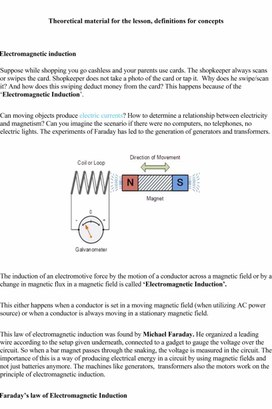PHY_10_64_V1_TG_Faraday law of induction.docx

Theoretical material for the lesson, definitions for concepts

Electromagnetic induction

Suppose while shopping you go cashless and your parents use cards. The shopkeeper always scans or swipes the card. Shopkeeper does not take a photo of the card or tap it.  Why does he swipe/scan it? And how does this swiping deduct money from the card? This happens because of the ‘Electromagnetic Induction’.

Can moving objects produce electric currents? How to determine a relationship between electricity and magnetism? Can you imagine the scenario if there were no computers, no telephones, no electric lights. The experiments of Faraday has led to the generation of generators and transformers.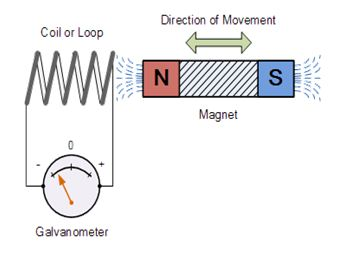The induction of an electromotive force by the motion of a conductor across a magnetic field or by a change in magnetic flux in a magnetic field is called ‘Electromagnetic Induction’.

This either happens when a conductor is set in a moving magnetic field (when utilizing AC power source) or when a conductor is always moving in a stationary magnetic field.

This law of electromagnetic induction was found by Michael Faraday. He organized a leading wire according to the setup given underneath, connected to a gadget to gauge the voltage over the circuit. So when a bar magnet passes through the snaking, the voltage is measured in the circuit. The importance of this is a way of producing electrical energy in a circuit by using magnetic fields and not just batteries anymore. The machines like generators,  transformers also the motors work on the principle of electromagnetic induction.

Faraday’s law of Electromagnetic Induction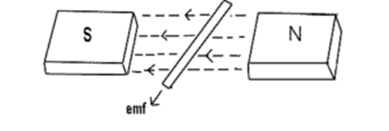Source: Electricaleasy

·         First law:  Whenever a conductor is placed in a varying magnetic field, EMF induces and this emf is called an induced emf and if the conductor is a closed circuit than the induced current flows through it.

·         Second law: The magnitude of the induced EMF is equal to the rate of change of flux linkages.

Based on his experiments we now have Faraday’s law of electromagnetic induction according to which the amount of voltage induced in a coil is proportional to the number of turns and the changing magnetic field of the coil.

So now, the induced voltage is as follows:

e = N × dΦdt

where,

e is the induced voltage
N is the number of turns in the coil
Φ is the magnetic flux
t is the time

Lenz’s law of Electromagnetic Induction

Lenz law of electromagnetic induction states that, when an emf induces according to Faraday’s law, the polarity (direction) of that induced emf is such that it opposes the cause of its production.

According to Lenz’s law

E = -N (dΦ/ dt)   (volts)

Eddy currents

By Lenz law of electromagnetic induction, the current swirls in such a way as to create a magnetic field opposing the change. Because of the tendency of eddy currents to oppose, eddy currents cause a loss of energy. Eddy currents transform more useful forms of energy, such as kinetic energy, into heat, which isn’t generally useful. In many applications, the loss of useful energy is not particularly desirable, but there are some practical applications. Like:

·         In the brakes of some trains. During braking, the brakes expose the metal wheels to a magnetic field which generates eddy currents in the wheels. The magnetic interaction between the applied field and the eddy currents slows the wheels down. The faster the wheels spin, the stronger is the effect, meaning that as the train slows the braking force is reduces, producing a smooth stopping motion.

·         There are few galvanometers having a fixed core which are of nonmagnetic metallic material. When the coil oscillates, the eddy currents that generate in the core oppose the motion and bring the coil to rest.

·         Induction furnace can be used to prepare alloys, by melting the metals. The eddy currents generated in the metals produce high temperature enough to melt it.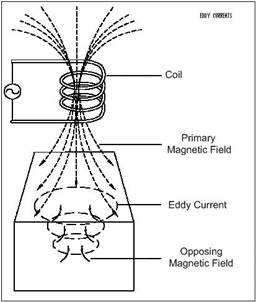Lesson starts with drawing magnetic field patterns around magnetic bars between different poles.

Then teacher ask what do you understand by Faraday’s law? Then would be discussion around answer to this question.

Then students can deduce topic of the lessen and objectives, for clarification you can show topic and the learning objectives on the presentation.

Then Subject-specific vocabulary & terminology will be presented to the students and their activities during the research work will be explained.

Then learners to investigate the effects of moving a bar magnet into and out of a coil connected to a sensitive centre-zero galvanometer. Teacher, discuss the results with the class and draw out the key points:

• There is an induced voltage in the (secondary) coil when the flux linkage changes.
• If a wire is moved parallel to the field lines there is no induced voltage.
• The size of the induced voltage depends on the rate of change of flux-linkage. (more obvious with the first experiment)
• The direction of the induced voltage (deflection of galvanometer) is such as to oppose the change causing it.

Flux-linkage can be changed by ‘flux-cutting (first experiment) or by flux-changing (second experiment). Then the animation is played to reinforce the conclusion drawn.

Here are video clips that could be used if apparatus is not available to carry out these experiments or else to review the results of the moving magnet experiment:

http://www.youtube.com/watch?v=hajIIGHPeuU

This longer video provides a more in depth survey of electromagnetic induction and goes further than is required but may be of interest to teachers and for use with able groups. Alternatively it can be used for the demonstrations only:

http://www.youtube.com/watch?annotation_id=annotation_595508&feature=iv&src_vid=hajIIGHPeuU&v=l-RjuauyuzM

This BBC bitesize page discusses Faraday’s Law:

http://www.bbc.co.uk/schools/gcsebitesize/science/add_ocr_pre_2011/electric_circuits/mainsproducedrev1.shtml

A helpful video showing how transformers work and discussing the underlying physics:

http://www.youtube.com/watch?v=ZjwzpoCiF8A

During each demonstration the learners are engaged in discussion using question and answer method. All learners engage in problem solving activity. Teacher guides learners through problem solving. Teacher demonstrates mutual induction using two coils placed one on top of the other. Show the pulse of induced voltage in a secondary coil when current is switched on and off through the primary coil. Students suggest how a continuous voltage could be generated in the secondary. Then demonstrate the effect using an A.C. supply connected to the primary and an oscilloscope connected to the secondary. During each demonstration the learners are engaged in discussion using question and answer method. Reinforce the LO’s. Answer any questions pupils may have/clarify understanding. Reflect on their learning.

Additional guidelines for organizing a lesson

Lesson starts with introducesing the topic of day and spells out the learning outcomes they will possess after the study. Acquaint students with the following issues:

The theme of the lesson

The objectives of the lesson

The criteria of success for the lesson

The plan of events for the lesson

Pre-teach the subject specific vocabulary.

Learners will share their experiences with electromagnetic induction.

Then students can deduce topic of the lessen and objectives, for clarification you can show topic and the learning objectives on the presentation.

Then Subject-specific vocabulary & terminology will be presented to the students and their activities during the research work will be explained.

Then teacher will ask learners to investigate the effects of moving a bar magnet into and out of a coil connected to a sensitive centre-zero galvanometer. After that would discuss the results with the class and draw out the key points:

-There is an induced voltage in the (secondary) coil when the flux linkage changes.

-If a wire is moved parallel to the field lines there is no induced voltage.

-The size of the induced voltage depends on the rate of change of flux-linkage. (more obvious with the first experiment)

-Induced current in a closed circuit counterwork the changes of a magnetic flux caused by this current.

Then teacher will explain Lenz’s law by using a magnet falling slowly in a copper tube by the existence of a magnetic field with opposite direction. Would give questions on a worksheet.

Learners demonstrate and explain Lenz’s law by using a magnet falling slowly in a copper tube by the existence of a magnetic field with opposite direction.

Watch videos on electromagnetic induction

Summarize their observations and conclusions findings.

Answers questions about the videos.

Attempt questions on the worksheet

Simulation of the ‘Faraday’s law’:

http://phet.colorado.edu/en/simulation/legacy/faraday

or

Simulation of the ‘Faraday’s law 2’:

http://phet.colorado.edu/en/simulation/faradays-law

The Faraday’s law by BBC:

http://www.bbc.co.uk/schools/gcsebitesize/science/add_ocr_pre_2011/electric_circuits/mainsproducedrev1.shtml

Lentz rule:

https://www.youtube.com/watch?v=M2e0JbIym-I&list=PLJ8TtQgi6QOqW-VQ0vB0XbzHz9_S8bh90&index=3

Lentz rule (experiment):

https://www.youtube.com/watch?v=q-Rd2DvlTU4

E.m.f induction in moving conductors:

https://www.youtube.com/watch?v=vGD4LWmAUv4&index=5&list=PLJ8TtQgi6QOqW-VQ0vB0XbzHz9_kS8bh90

Then teacher Highlight key concepts, definitions, and equations learnt using the concept map, ask students to do questions on the worksheet provided, look forward to the next lesson.

During this time students attempt the questions given by the teacher, summarize the main concepts, definitions, and equations learnt, reflect on their own learning, evaluate their own work and the work of their classmates. Teacher will give homework complete the specified thinking tasks for this lesson.

Additional multilevel (on differentiation) tasks

The teacher  assigns questions 1- 15  to weak students, questions 16- 26 to the average students, questions 27-34 to the strong students

Recommendations for formative assessment

Students think of everything they already know about the importance of electricity for our life, and record this information in the first column of the table given.

Students discuss learning objectives and success criteria.

Students work in groups. Each group fill out one line of the table provided by the teacher.

Students share their knowledge with neighboring pairs, filling in the table. Students make a list of electric charge properties significant for our daily life.

Answers, criteria for assignments, additional materials for the lesson

Exam Solutions

1.      emf stands for electromotive force. It is a potential difference applied to a full circuit.

2.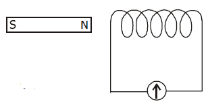Electromagnetic Induction occurs when an emf is induced in a coil due to a changing magnetic flux.

3.      Apparatus: coil, magnet and galvanometer.

Procedure: Set up as shown.

Move the magnet in and out of the coil.

Observation: the needle deflects.

4.

(i)     The needle in the galvanometer deflects.

(ii)  An emf is induced in the coil of wire, which in turn produces a current which moves the needle.

(iii)            There is a greater deflection of the needle.

5.

(i)     Correct diagram to include magnet, coil and meter

(ii)  Electromagnetic induction

(iii)            Kinetic to electric

(iv) Current stopped whenever the magnet was motionless // electricity is only generated when the magnet or coil is moving.

6.      Faraday’s Law states that the size of the induced emf is proportional to the rate of change of flux.

7.      Lenz’s Law states that the direction of the induced emf is always such as to oppose the change producing it.

8.      Faraday’s Law states that the size of the induced emf is proportional to the rate of change of flux.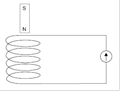Lenz’s Law states that the direction of the induced emf is always such as to oppose the change producing it.

9.      Set up as shown.

Move the magnet in and out of the coil and note the deflection in the galvanometer.

10.  Move the magnet in and out of the coil slowly and note a slight deflection.

Move the magnet quickly and note a greater deflection.

11.

(i)     Current flows in the ring in such a direction as to oppose the change which caused it. Therefore the ring follows the magnet.

(ii)  The ring would be repelled.

12.

(i)     An emf is induced in the copper because is its experiencing a changing magnetic field.

This produces a current.

This current has a magnetic field associated with it which opposes the motion of the magnet.

(ii)  Kinetic (or potential) to electrical (or heat).

13.

(i)     Alternating current.

(ii)  There is no longer a full circuit, so even though there is an induced potential difference there is no (induced) current, therefore no induced magnetic field in the coil therefore no opposing force.

14.  Magnetic flux is defined as the product of magnetic flux density and area.

(i)     E=−N(Final Flux –Initial Flux) / time taken

E = 5000(8 × 10-4 /0.1) = 40 V

(ii)  E= N [(Final Flux –Initial Flux) / time taken]  = (20)[(2.3 – 1.4)/0.4]

E = 45 V

15.

(iii)            t = dist/velocity = 5 cm / 500 cm s-1 = 0.01 s

(iv) Φ = BA = (8)(.05 × .05) = 0.02 webers.

(v)   Induced emf = (Final Flux –Initial Flux) / Time Taken

= (0 - 0.02)/0.01

= 2 Volts

16.

(i)     A = (0.05)2 = 0.0025

φ (= BA ) = (4)(0.0025)

φ = 0.01 Wb

(ii)  E = N(Δφ/Δt)

Δφ /Δt = (0.01 – 0 )/0.2  = 0.05

E= 200(0.05)  E = 10 V

Because only metal strings can be magnitised.

(a)   Because the induced emf varies due to the amplitude of the vibrating string.

(b)   A  louder sound is produced.

17.  V max = (√2)(Vrms)             Vrms= 325/√2  =  230 V

18.  Labelled axes: time on the horizontal and voltage on the vertical.

19.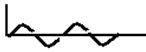Alternating current

20.  (i) axes labelled (I and t),sinusoidal curve (at least one full wave)

(ii) (axes labelled) correct curve

21.  A diode is a device that allows current to flow in one direction only.

22.  Radio, television, computer, battery charger, mobile phone charger.

23.  Dynamo, generator, induction motor, transformer, dynamo

24.  To increase or decrease voltage.

25.  Computer, radio, TV, doorbell, washing machine, mobile phone chargers, power supply, etc.

26.  A = primary / input coil, B = secondary / output coil, C = iron core

27.   It has a laminated core.

28.   10% of the power in is lost.

29.  Kinetic to electric.

30.  Vin/Vout = Ninput/Noutput

230/Vout = 400/100

Vout = 230 × (100/400) = 57.5 Volts

31.  Vin/Vout = Ninput/Noutput

230/Vout = 400/1200

Vout = 230 × (400/1200) = 690 Volts

32.  Vin/Vout = Ninput/Noutput

230/Vout = 4600/120

Vout = 230 × (120/4600) = 6 Volts

33.

(i)     Current is reduced

(ii)  An emf is induced in the coil which induces a current which opposes the initial current.

34.

(i)     There would normally be a back emf in the coil due to the alternating current  being supplied.

When the core was inserted it increased the magnetic flux which in turn increased the self-induction (back emf) and this reduced the overall voltage and therefore the overall current.

(ii)  Dimmer switch, smooth d.c., tuning radios, braking trains, damping in balances, induction coil

Скачано с www.znanio.ru

## Theoretical material for the lesson, definitions for concepts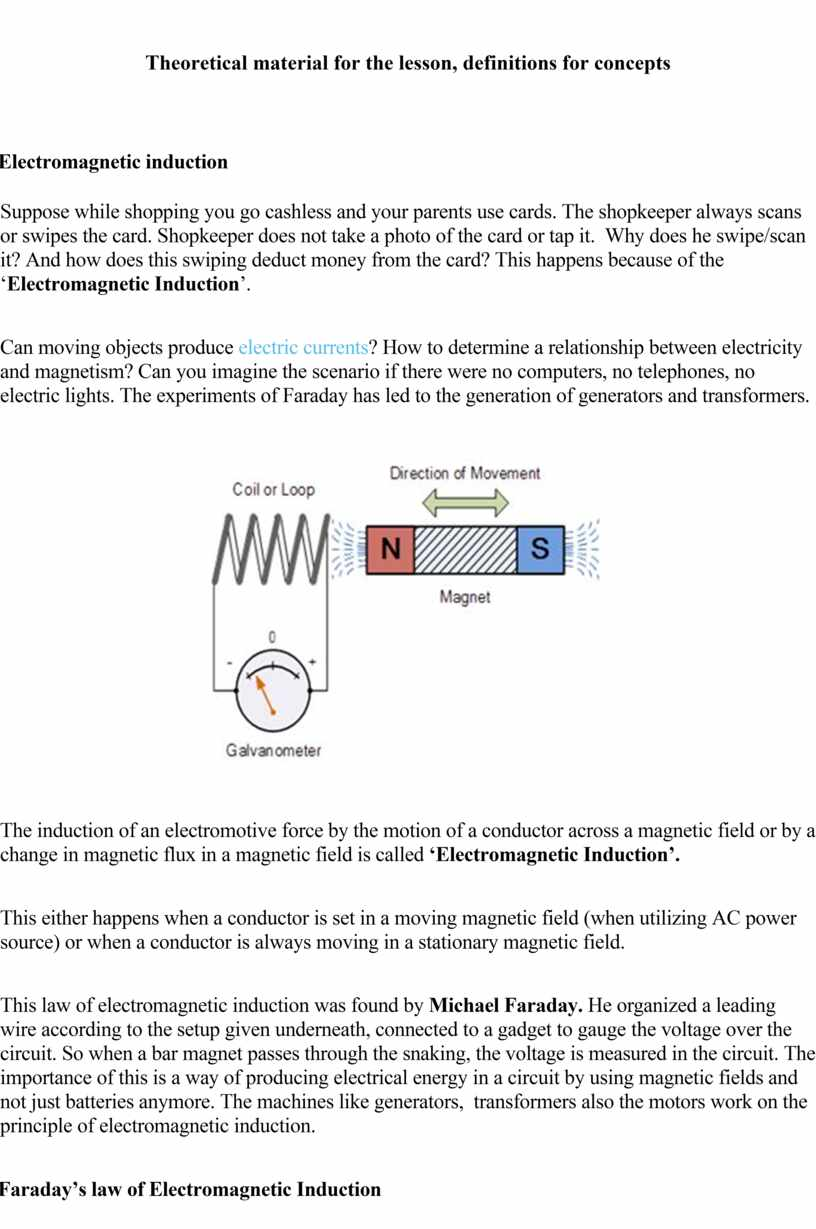## In the brakes of some trains. During braking, the brakes expose the metal wheels to a magnetic field which generates eddy currents in the wheels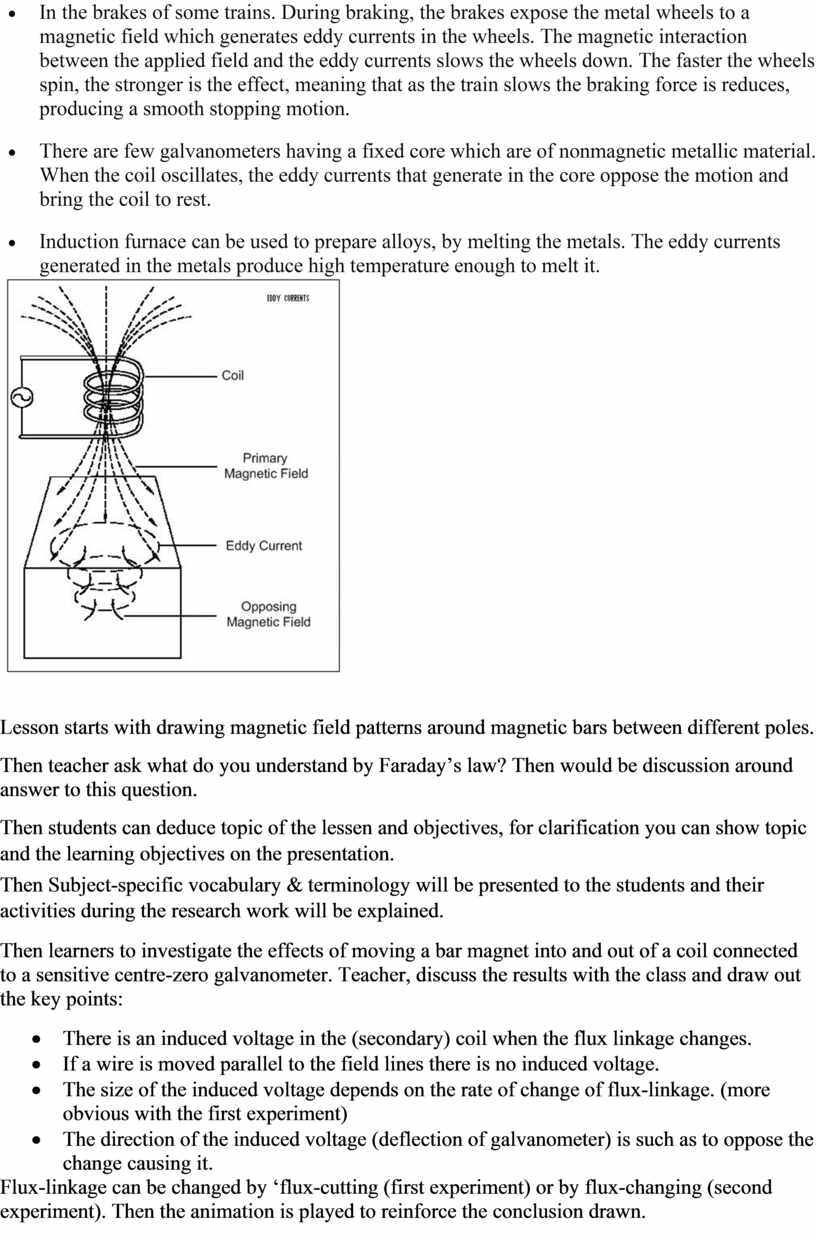## Here are video clips that could be used if apparatus is not available to carry out these experiments or else to review the results of…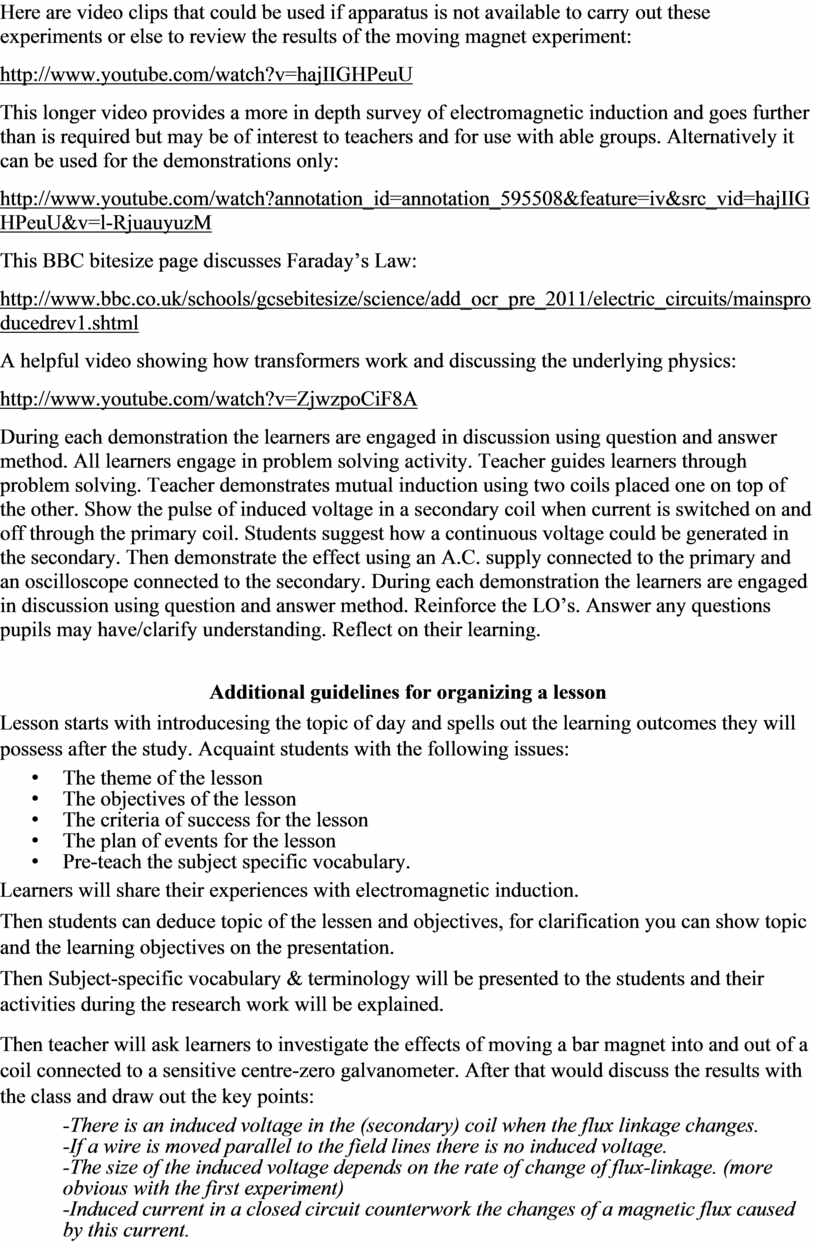## Then teacher will explain Lenz’s law by using a magnet falling slowly in a copper tube by the existence of a magnetic field with opposite…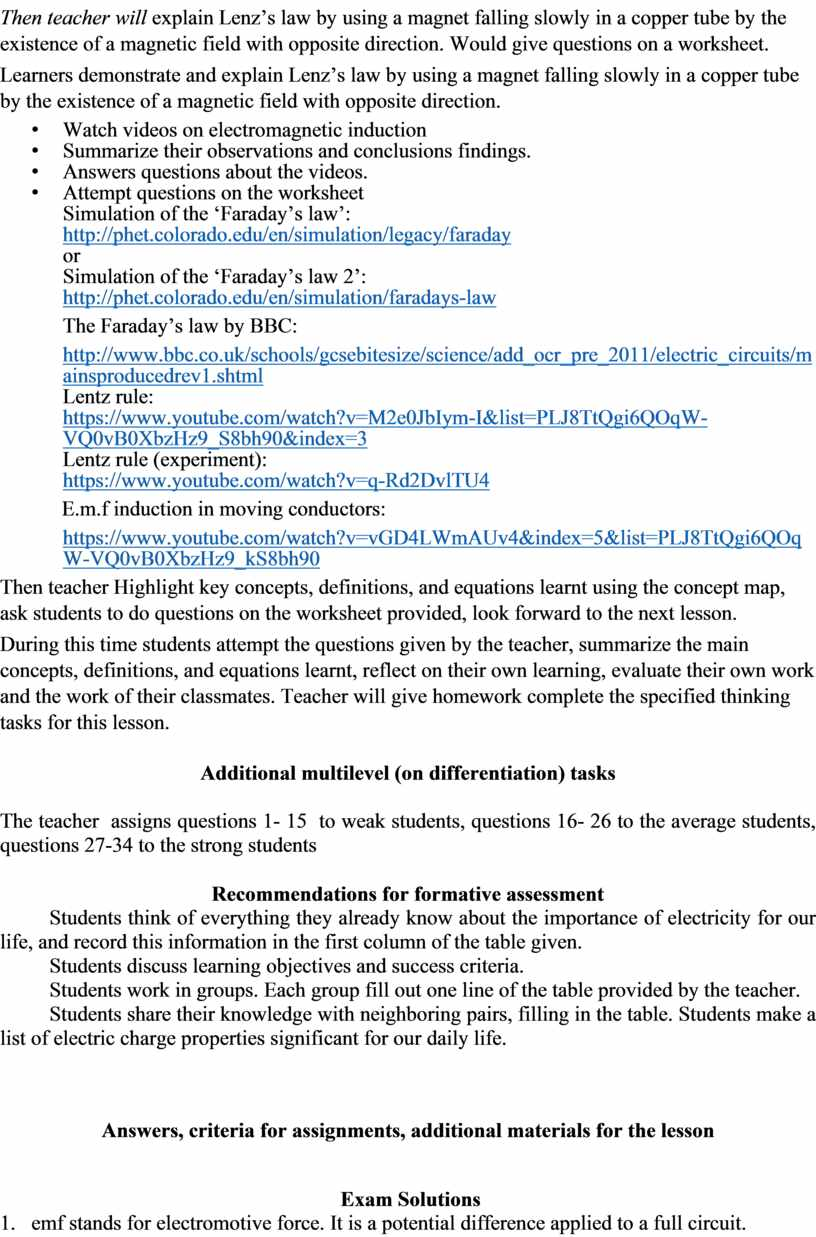## Electromagnetic Induction occurs when an emf is induced in a coil due to a changing magnetic flux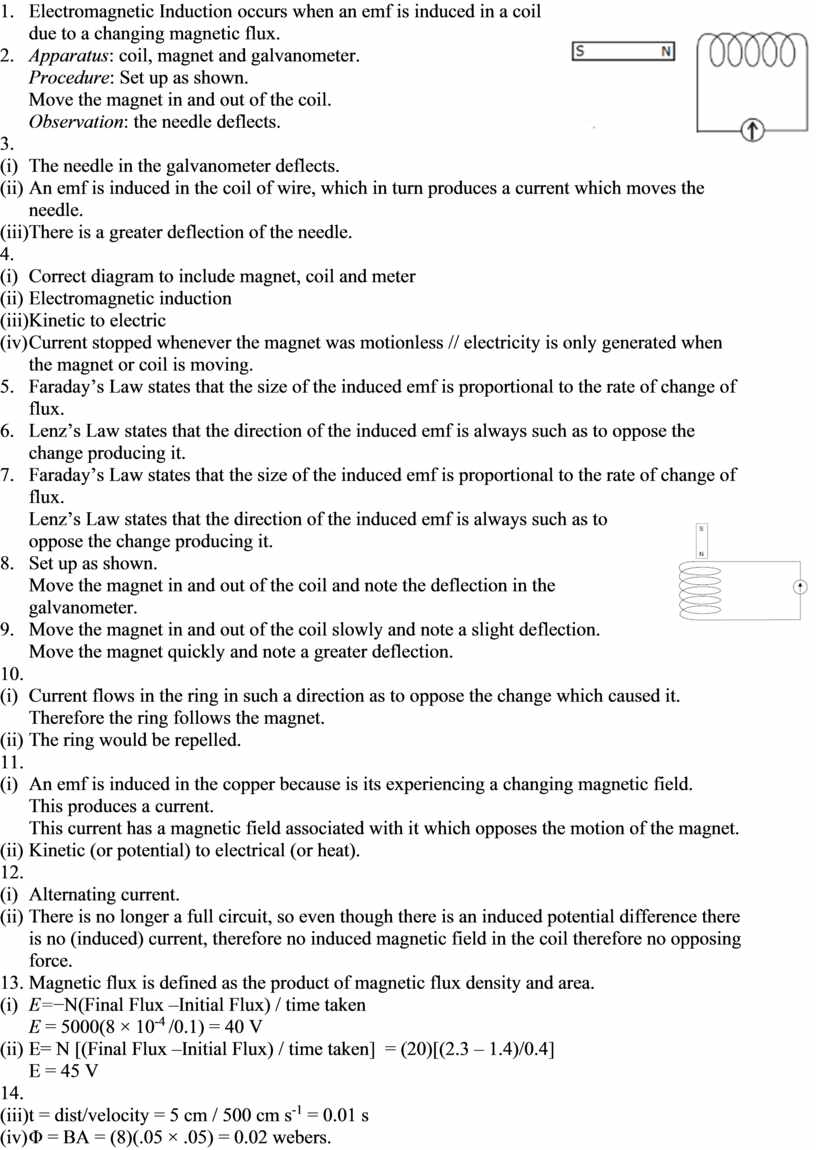Скачать файл